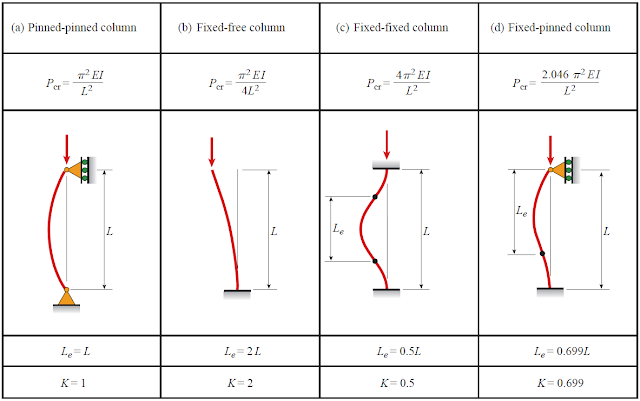# Failure in Columns

Columns can be classified as three types: short, long, and intermediate. Failure in short columns is distinguished by the crushing (yielding) of material, due to the stresses developed in the material which are greater than the yielding stress. Long column fails are due to buckling, meaning that the column actually defects laterally and bends near the middle of its length. Buckling in long columns occurs at stresses below the yielding stress of the material. Failure in intermediate columns is a combination of material yielding and buckling. Buckling in intermediate columns takes place at higher stresses.
Leonhard Euler (1707–1783), Swiss mathematician, derived a formula for a slender column that shows how the critical load would cause buckling in this type of column. The formula is the Euler equation and was named after him for his great contribution to column theory. Today it is still used as the basis for the analysis and design of slender columns. The Euler equation is expressed as
Pe =  π2EI/Le2

where
Pe = the critical load or the Euler buckling load, lb or N.
E = the modulus of elasticity of the material, psi or MPa
I = the smallest moment of inertia of the cross section, in.4 or mm4
Le = the length of the column between the hinges, in. or mm

The Euler formula is used to precisely predict the buckling load if the buckling stress is less than the proportional limit of the material. For the purpose of comparison, the Euler formula can be expressed in terms of buckling stress using and substituting Ar2 for the moment of inertia, I.

Pe/A =  (π2EI)/(Le/r)2

or
σe =  (π2EI)/(Le/r)2

The Euler equation has some limitations in the fact that it is only used if buckling occurs, as stresses on a column are low and in the material’s elastic region. The length between the hinges also known as buckling length varies with different support conditions.Critical loads, effective lengths and effective-length factors for ideal columnsBuckling lengths of columns for different support conditions